Deep learning with Python 学习笔记（10）

生成式深度学习

使用 LSTM 生成文本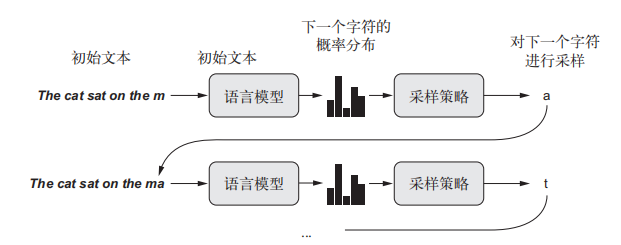import numpy as np
def reweight_distribution(original_distribution, temperature=0.5):
#original_distribution 是概率值组成的一维 Numpy 数组，这些概率值之和必须等于 1。temperature 是一个因子，用于定量描述输出分布的熵
distribution = np.log(original_distribution) / temperature
distribution = np.exp(distribution)
return distribution / np.sum(distribution)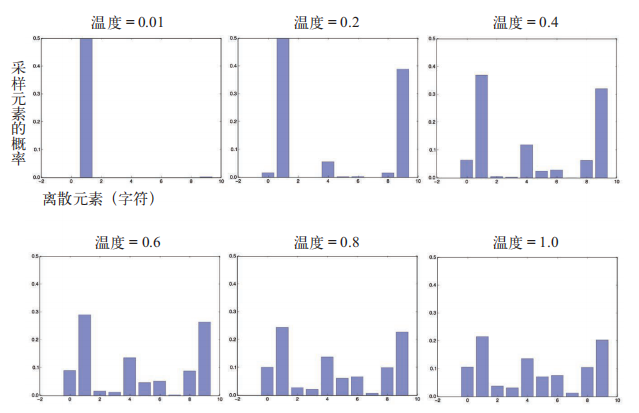1. 给定目前已生成的文本，从模型中得到下一个字符的概率分布
2. 根据某个温度对分布进行重新加权
3. 根据重新加权后的分布对下一个字符进行随机采样
4. 将新字符添加到文本末尾

demo

import keras
import numpy as np
from keras import layers
import random
import sys

path = keras.utils.get_file('nietzsche.txt', origin='https://s3.amazonaws.com/text-datasets/nietzsche.txt')
# 将语料转为小写
print('Corpus length:', len(text))

maxlen = 60
step = 3
sentences = []
next_chars = []
for i in range(0, len(text) - maxlen, step):
sentences.append(text[i: i + maxlen])
next_chars.append(text[i + maxlen])
print('Number of sequences:', len(sentences))
# 语料中唯一字符组成的列表
chars = sorted(list(set(text)))
print('Unique characters:', len(chars))
# 将唯一字符映射为它在列表 chars 中的索引
char_indices = dict((char, chars.index(char)) for char in chars)

print('Vectorization...')
# 将字符 one-hot 编码为二进制数组
x = np.zeros((len(sentences), maxlen, len(chars)), dtype=np.bool)
y = np.zeros((len(sentences), len(chars)), dtype=np.bool)
for i, sentence in enumerate(sentences):
for t, char in enumerate(sentence):
x[i, t, char_indices[char]] = 1
y[i, char_indices[next_chars[i]]] = 1

# 用于预测下一个字符的单层 LSTM 模型
model = keras.models.Sequential()

optimizer = keras.optimizers.RMSprop(lr=0.01)
model.compile(loss='categorical_crossentropy', optimizer=optimizer)

# 模型预测，采样下一个字符的函数
def sample(preds, temperature=1.0):
preds = np.asarray(preds).astype('float64')
preds = np.log(preds) / temperature
exp_preds = np.exp(preds)
preds = exp_preds / np.sum(exp_preds)
probas = np.random.multinomial(1, preds, 1)
return np.argmax(probas)

for epoch in range(1, 41):
print('epoch', epoch)
model.fit(x, y, batch_size=128, epochs=1)
start_index = random.randint(0, len(text) - maxlen - 1)
generated_text = text[start_index: start_index + maxlen]
print('--- Generating with seed: "' + generated_text + '"')
for temperature in [0.2, 0.5, 1.0, 1.2]:
print('------ temperature:', temperature)
sys.stdout.write(generated_text)
for i in range(400):
sampled = np.zeros((1, maxlen, len(chars)))
for t, char in enumerate(generated_text):
sampled[0, t, char_indices[char]] = 1.
preds = model.predict(sampled, verbose=0)
next_index = sample(preds, temperature)
next_char = chars[next_index]
generated_text += next_char
generated_text = generated_text[1:]
sys.stdout.write(next_char)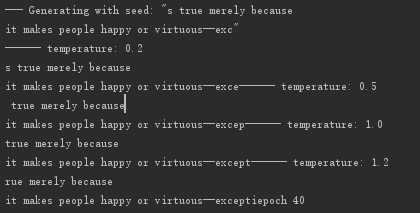DeepDream

DeepDream 是一种艺术性的图像修改技术，它用到了卷积神经网络学到的表示。DeepDream 算法与的卷积神经网络过滤器可视化技术几乎相同，都是反向运行一个卷积神经网络：对卷积神经网络的输入做梯度上升，以便将卷积神经网络靠顶部的某一层的某个过滤器激活最大化。DeepDream 使用了相同的想法，但有以下这几个简单的区别

1. 使用 DeepDream，我们尝试将所有层的激活最大化，而不是将某一层的激活最大化，因此需要同时将大量特征的可视化混合在一起
2. 不是从空白的、略微带有噪声的输入开始，而是从现有的图像开始，因此所产生的效果能够抓住已经存在的视觉模式，并以某种艺术性的方式将图像元素扭曲
3. 输入图像是在不同的尺度上［叫作八度（octave）］进行处理的，这可以提高可视化的质量

DeepDream 过程：空间处理尺度的连续放大（八度）与放大时重新注入细节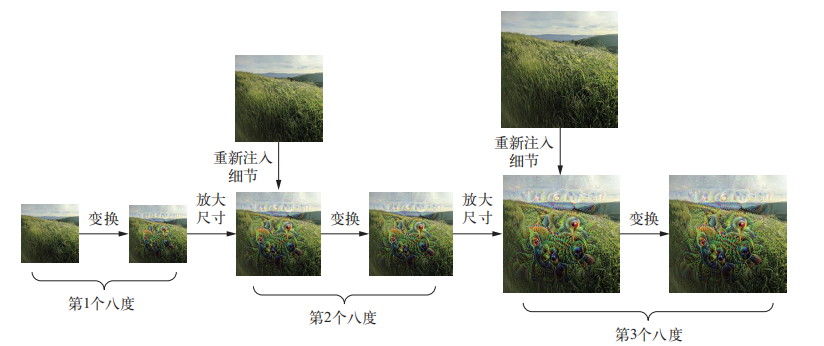1. 加载预训练的 Inception V3 模型
2. 计算损失（loss），即在梯度上升过程中需要最大化的量

1. (2)设置 DeepDream 配置
2. (2)定义需要最大化的损失
3. 设置梯度上升过程
4. 在多个连续尺度上运行梯度上升

demo

from keras.applications import inception_v3
from keras import backend as K
import numpy as np
import scipy
from keras.preprocessing import image

def resize_img(img, size):
img = np.copy(img)
factors = (1, float(size) / img.shape, float(size) / img.shape, 1)
return scipy.ndimage.zoom(img, factors, order=1)

def save_img(img, fname):
pil_img = deprocess_image(np.copy(img))
scipy.misc.imsave(fname, pil_img)

def preprocess_image(image_path):
img = image.img_to_array(img)
img = np.expand_dims(img, axis=0)
img = inception_v3.preprocess_input(img)
return img

def deprocess_image(x):
if K.image_data_format() == 'channels_first':
x = x.reshape((3, x.shape, x.shape))
x = x.transpose((1, 2, 0))
else:
x = x.reshape((x.shape, x.shape, 3))
x /= 2.
x += 0.5
x *= 255.
x = np.clip(x, 0, 255).astype('uint8')
return x

# 这个命令会禁用所有与训练有关的操作
K.set_learning_phase(0)
# 构建不包括全连接层的 Inception V3网络。使用预训练的 ImageNet 权重来加载模型
model = inception_v3.InceptionV3(weights='imagenet', include_top=False)

# 将层的名称映射为一个系数，这个系数定量表示该层激活对你要最大化的损失的贡献大小
layer_contributions = {
'mixed2': 0.2,
'mixed3': 3.,
'mixed4': 2.,
'mixed5': 1.5,
}

# 定义最大化的损失
layer_dict = dict([(layer.name, layer) for layer in model.layers])
loss = K.variable(0.)
for layer_name in layer_contributions:
coeff = layer_contributions[layer_name]
activation = layer_dict[layer_name].output
scaling = K.prod(K.cast(K.shape(activation), 'float32'))
# 将该层特征的L2范数添加到loss中。为了避免出现边界伪影，损失中仅包含非边界的像素
loss += coeff * K.sum(K.square(activation[:, 2: -2, 2: -2, :])) / scaling

# 梯度上升过程
# 这个张量用于保存生成的图像，即梦境图像
dream = model.input
# 计算损失相对于梦境图像的梯度
# 将梯度标准化
# 给定一张输出图像，设置一个 Keras 函数来获取损失值和梯度值

loss_value = outs

# 运行 iterations次梯度上升
for i in range(iterations):
if max_loss is not None and loss_value > max_loss:
break
print('...Loss value at', i, ':', loss_value)
return x

# 　在多个连续尺度上运行梯度上升
# 梯度上升的步长
step = 0.01
# 运行梯度上升的尺度个数
num_octave = 3
# 两个尺度之间的大小比例
octave_scale = 1.4
iterations = 20
# 如果损失增大到大于 10，我们要中断梯度上升过程，以避免得到丑陋的伪影
max_loss = 10.
base_image_path = 'img_url'
img = preprocess_image(base_image_path)
original_shape = img.shape[1:3]
successive_shapes = [original_shape]
for i in range(1, num_octave):
# 一个由形状元组组成的列表，它定义了运行梯度上升的不同尺度
shape = tuple([int(dim / (octave_scale ** i)) for dim in original_shape])
successive_shapes.append(shape)
# 将形状列表反转，变为升序
successive_shapes = successive_shapes[::-1]
original_img = np.copy(img)
# 将图像 Numpy 数组的大小缩放到最小尺寸
shrunk_original_img = resize_img(img, successive_shapes)
for shape in successive_shapes:
print('Processing image shape', shape)
# 将梦境图像放大
img = resize_img(img, shape)
# 运行梯度上升，改变梦境图像
step=step, max_loss=max_loss)
# 将原始图像的较小版本放大，它会变得像素化
upscaled_shrunk_original_img = resize_img(shrunk_original_img, shape)
# 在这个尺寸上计算原始图像的高质量版本
same_size_original = resize_img(original_img, shape)
lost_detail = same_size_original - upscaled_shrunk_original_img
# 将丢失的细节重新注入到梦境图像中
img += lost_detail
shrunk_original_img = resize_img(original_img, shape)
save_img(img, fname='dream_at_scale_' + str(shape) + '.png')
save_img(img, fname='final_dream.png')dreamImage神经风格迁移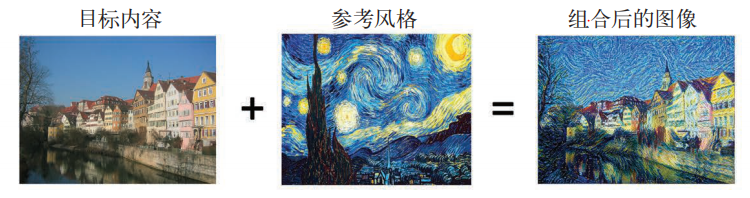loss = distance(style(reference_image) - style(generated_image)) + distance(content(original_image) - content(generated_image))

1. 在目标内容图像和生成图像之间保持相似的较高层激活，从而能够保留内容。卷积神经网络应该能够“看到”目标图像和生成图像包含相同的内容
2. 在较低层和较高层的激活中保持类似的相互关系（correlation），从而能够保留风格。特征相互关系捕捉到的是纹理（texture），生成图像和风格参考图像在不同的空间尺度上应该具有相同的纹理

1. 创建一个网络，它能够同时计算风格参考图像、目标图像和生成图像的 VGG19 层激活
2. 使用这三张图像上计算的层激活来定义之前所述的损失函数，为了实现风格迁移，需要将这个损失函数最小化
3. 设置梯度下降过程来将这个损失函数最小化

demo

import numpy as np
from keras.applications import vgg19
from keras import backend as K
from scipy.optimize import fmin_l_bfgs_b
from scipy.misc import imsave
import time

def preprocess_image(image_path):
img = img_to_array(img)
img = np.expand_dims(img, axis=0)
img = vgg19.preprocess_input(img)
return img

def deprocess_image(x):
# vgg19.preprocess_input 的作用是减去 ImageNet 的平均像素值，
# 使其中心为 0。这里相当于 vgg19.preprocess_input 的逆操作
x[:, :, 0] += 103.939
x[:, :, 1] += 116.779
x[:, :, 2] += 123.68
# 将图像由 BGR 格式转换为 RGB 格式。这也是
# vgg19.preprocess_input 逆操作的一部分
x = x[:, :, ::-1]
x = np.clip(x, 0, 255).astype('uint8')
return x

target_image_path = 'cat.jpg'
style_reference_image_path = 'style.png'

# 设置生成图像的尺寸
img_height = 400
img_width = int(width * img_height / height)
# 加载预训练的 VGG19 网络，并将其应用于三张图像
target_image = K.constant(preprocess_image(target_image_path))
style_reference_image = K.constant(preprocess_image(style_reference_image_path))
# 占位符用于保存生成图像
combination_image = K.placeholder((1, img_height, img_width, 3))
# 将三张图像合并为一个批量
input_tensor = K.concatenate([target_image, style_reference_image, combination_image], axis=0)
model = vgg19.VGG19(input_tensor=input_tensor, weights='imagenet', include_top=False)

def content_loss(base, combination):
"""
内容损失
:param base:
:param combination:
:return:
"""
return K.sum(K.square(combination - base))

def gram_matrix(x):
features = K.batch_flatten(K.permute_dimensions(x, (2, 0, 1)))
gram = K.dot(features, K.transpose(features))
return gram

def style_loss(style, combination):
"""
风格损失
:param style:
:param combination:
:return:
"""
S = gram_matrix(style)
C = gram_matrix(combination)
channels = 3
size = img_height * img_width
return K.sum(K.square(S - C)) / (4. * (channels ** 2) * (size ** 2))

def total_variation_loss(x):
"""
总变差损失
对生成的组合图像的像素进行操作，
促使生成图像具有空间连续性，从而避免结果过度像素化
也可以简单理解为正则化损失
:param x:
:return:
"""
a = K.square(x[:, :img_height - 1, :img_width - 1, :] - x[:, 1:, :img_width - 1, :])
b = K.square(x[:, :img_height - 1, :img_width - 1, :] - x[:, :img_height - 1, 1:, :])
return K.sum(K.pow(a + b, 1.25))

# 定义最小化的最终损失
# 将层的名称映射为激活张量的字典
outputs_dict = dict([(layer.name, layer.output) for layer in model.layers])
content_layer = 'block5_conv2'
style_layers = ['block1_conv1', 'block2_conv1', 'block3_conv1', 'block4_conv1', 'block5_conv1']
total_variation_weight = 1e-4
style_weight = 1.
content_weight = 0.025
loss = K.variable(0.)
layer_features = outputs_dict[content_layer]
target_image_features = layer_features[0, :, :, :]
combination_features = layer_features[2, :, :, :]
loss += content_weight * content_loss(target_image_features, combination_features)
for layer_name in style_layers:
layer_features = outputs_dict[layer_name]
style_reference_features = layer_features[1, :, :, :]
combination_features = layer_features[2, :, :, :]
sl = style_loss(style_reference_features, combination_features)
loss += (style_weight / len(style_layers)) * sl
loss += total_variation_weight * total_variation_loss(combination_image)

# 使用 L-BFGS 算法进行优化,设置梯度下降过程
# 获取损失相对于生成图像的梯度
# 用于获取当前损失值和当前梯度值的函数

class Evaluator(object):
"""
装起来，让你可以利用两个单独的方法
调用来获取损失和梯度，这是我们要使
用的 SciPy 优化器所要求的
"""
def __init__(self):
self.loss_value = None

def loss(self, x):
assert self.loss_value is None
x = x.reshape((1, img_height, img_width, 3))
loss_value = outs
self.loss_value = loss_value
return self.loss_value

assert self.loss_value is not None
self.loss_value = None

evaluator = Evaluator()

# 使用 SciPy 的 L-BFGS 算法来运行梯度上升过程
# 风格迁移循环
result_prefix = 'my_result'
iterations = 20
x = preprocess_image(target_image_path)
# 将图像展平，因为 scipy.optimize.fmin_l_bfgs_b 只能处理展平的向量
x = x.flatten()
for i in range(iterations):
print('Start of iteration', i)
start_time = time.time()
x, min_val, info = fmin_l_bfgs_b(evaluator.loss, x, fprime=evaluator.grads, maxfun=20)
print('Current loss value:', min_val)
# 保存当前的生成图像
img = x.copy().reshape((img_height, img_width, 3))
img = deprocess_image(img)
fname = result_prefix + '_at_iteration_%d.png' % i
imsave(fname, img)
print('Image saved as', fname)
end_time = time.time()
print('Iteration %d completed in %ds' % (i, end_time - start_time))

用变分自编码器生成图像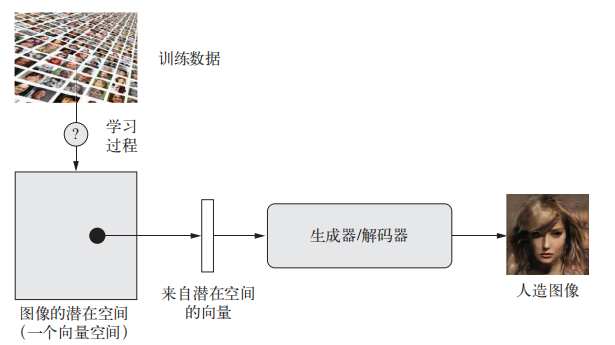VAE 生成的人脸连续空间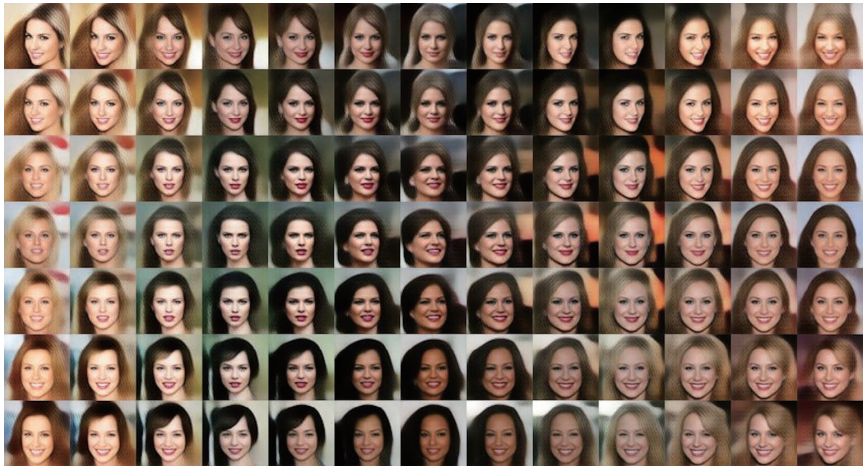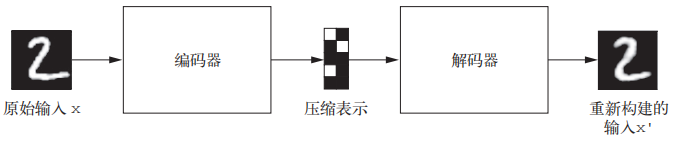VAE 不是将输入图像压缩成潜在空间中的固定编码，而是将图像转换为统计分布的参数，即平均值和方差。本质上来说，这意味着我们假设输入图像是由统计过程生成的，在编码和解码过程中应该考虑这一过程的随机性。然后，VAE 使用平均值和方差这两个参数来从分布中随机采样一个元素，并将这个元素解码到原始输入。这个过程的随机性提高了其稳健性，并迫使潜在空间的任何位置都对应有意义的表示，即潜在空间采样的每个点都能解码为有效的输出

VAE模型表示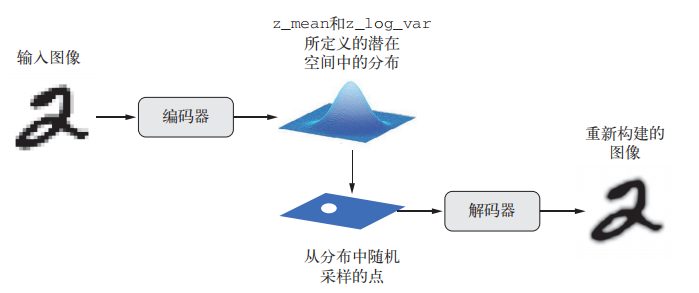VAE 的工作原理

1. 一个编码器模块将输入样本 input_img 转换为表示潜在空间中的两个参数 z_mean 和 z_log_variance
2. 我们假定潜在正态分布能够生成输入图像，并从这个分布中随机采样一个点 z：z = z_mean + exp(z_log_variance) * epsilon，其中 epsilon 是取值很小的随机张量
3. 一个解码器模块将潜在空间的这个点映射回原始输入图像

VAE 的参数通过两个损失函数来进行训练：一个是重构损失（reconstruction loss），它迫使解码后的样本匹配初始输入；另一个是正则化损失（regularization loss），它有助于学习具有良好结构的潜在空间，并可以降低在训练数据上的过拟合

demo

import keras
from keras import layers
from keras import backend as K
from keras.models import Model
import numpy as np
from keras.datasets import mnist
import matplotlib.pyplot as plt
from scipy.stats import norm

class CustomVariationalLayer(keras.layers.Layer):
"""
用于计算 VAE 损失的自定义层
"""
def vae_loss(self, x, z_decoded):
x = K.flatten(x)
z_decoded = K.flatten(z_decoded)
xent_loss = keras.metrics.binary_crossentropy(x, z_decoded)
kl_loss = -5e-4 * K.mean(1 + z_log_var - K.square(z_mean) - K.exp(z_log_var), axis=-1)
return K.mean(xent_loss + kl_loss)

def call(self, inputs):
x = inputs
z_decoded = inputs
loss = self.vae_loss(x, z_decoded)
return x

def sampling(args):
"""
潜在空间采样的函数
:param args:
:return:
"""
z_mean, z_log_var = args
epsilon = K.random_normal(shape=(K.shape(z_mean), latent_dim),
mean=0., stddev=1.)
return z_mean + K.exp(z_log_var) * epsilon

# 网络
img_shape = (28, 28, 1)
batch_size = 16
latent_dim = 2
input_img = keras.Input(shape=img_shape)
x = layers.Conv2D(32, 3, padding='same', activation='relu')(input_img)
x = layers.Conv2D(64, 3, padding='same', activation='relu', strides=(2, 2))(x)
x = layers.Conv2D(64, 3, padding='same', activation='relu')(x)
x = layers.Conv2D(64, 3, padding='same', activation='relu')(x)
shape_before_flattening = K.int_shape(x)
x = layers.Flatten()(x)
x = layers.Dense(32, activation='relu')(x)
z_mean = layers.Dense(latent_dim)(x)
z_log_var = layers.Dense(latent_dim)(x)

# z_mean 和 z_log_var 是统计分布的参数，假设这个分布能够生成 input_img
# 接下来的代码将使用 z_mean 和 z_log_var 来生成一个潜在空间点 z
z = layers.Lambda(sampling)([z_mean, z_log_var])
# VAE 解码器网络，将潜在空间点映射为图像
decoder_input = layers.Input(K.int_shape(z)[1:])
# 对输入进行上采样
x = layers.Dense(np.prod(shape_before_flattening[1:]), activation='relu')(decoder_input)
# 将 z 转换为特征图，使其形状与编码器模型最后一个 Flatten 层之前的特征图的形状相同
x = layers.Reshape(shape_before_flattening[1:])(x)
# 使用一个 Conv2DTranspose 层和一个Conv2D 层，将 z 解码为与原始输入图像具有相同尺寸的特征图
x = layers.Conv2DTranspose(32, 3, padding='same', activation='relu', strides=(2, 2))(x)
x = layers.Conv2D(1, 3, padding='same', activation='sigmoid')(x)
# 将解码器模型实例化，它将 decoder_input转换为解码后的图像
decoder = Model(decoder_input, x)
# 将实例应用于 z，以得到解码后的 z
z_decoded = decoder(z)

y = CustomVariationalLayer()([input_img, z_decoded])

# 训练 VAE
vae = Model(input_img, y)
vae.compile(optimizer='rmsprop', loss=None)
vae.summary()
(x_train, _), (x_test, y_test) = mnist.load_data()
x_train = x_train.astype('float32') / 255.
x_train = x_train.reshape(x_train.shape + (1,))
x_test = x_test.astype('float32') / 255.
x_test = x_test.reshape(x_test.shape + (1,))
vae.fit(x=x_train, y=None, shuffle=True, epochs=10, batch_size=batch_size, validation_data=(x_test, None))

# 从二维潜在空间中采样一组点的网格，并将其解码为图像
n = 15
digit_size = 28
figure = np.zeros((digit_size * n, digit_size * n))
grid_x = norm.ppf(np.linspace(0.05, 0.95, n))
grid_y = norm.ppf(np.linspace(0.05, 0.95, n))

for i, yi in enumerate(grid_x):
for j, xi in enumerate(grid_y):
z_sample = np.array([[xi, yi]])
z_sample = np.tile(z_sample, batch_size).reshape(batch_size, 2)
x_decoded = decoder.predict(z_sample, batch_size=batch_size)
digit = x_decoded.reshape(digit_size, digit_size)
figure[i * digit_size: (i + 1) * digit_size, j * digit_size: (j + 1) * digit_size] = digit
plt.figure(figsize=(10, 10))
plt.imshow(figure, cmap='Greys_r')
plt.show()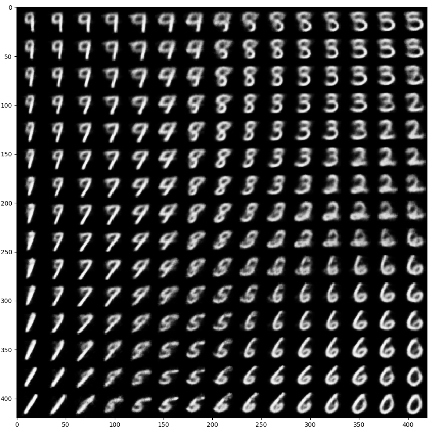VAE 得到的是高度结构化的、连续的潜在表示。因此，它在潜在空间中进行各种图像编辑的效果很好，比如换脸、将皱眉脸换成微笑脸等。它制作基于潜在空间的动画效果也很好，比如沿着潜在空间的一个横截面移动，从而以连续的方式显示从一张起始图像缓慢变化为不同图像的效果
GAN 可以生成逼真的单幅图像，但得到的潜在空间可能没有良好的结构，也没有很好的连续性

GAN 的工作原理：一个伪造者网络和一个专家网络，二者训练的目的都是为了打败彼此。因此，GAN 由以下两部分组成

GAN示意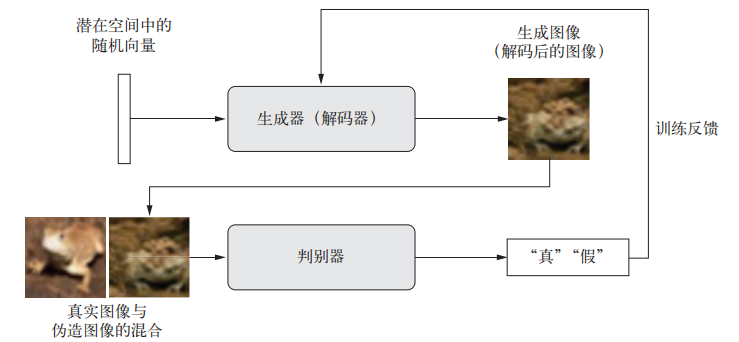GAN系统的优化最小值是不固定的。通常来说，梯度下降是沿着静态的损失地形滚下山坡。但对于 GAN 而言，每下山一步，都会对整个地形造成一点改变。它是一个动态的系统，其最优化过程寻找的不是一个最小值，而是两股力量之间的平衡。因此，GAN 的训练极其困难，想要让 GAN 正常运行，需要对模型架构和训练参数进行大量的仔细调整

GAN 的简要实现流程

1. generator网络将形状为(latent_dim,)的向量映射到形状为(32, 32, 3)的图像
2. discriminator 网络将形状为 (32, 32, 3) 的图像映射到一个二进制分数，用于评估图像为真的概率
3. gan 网络将 generator 网络和 discriminator 网络连接在一起：gan(x) = discriminator(generator(x))。生成器将潜在空间向量解码为图像，判别器对这些图像的真实性进行评估，因此这个 gan 网络是将这些潜在向量映射到判别器的评估结果
4. 我们使用带有“真”/“假”标签的真假图像样本来训练判别器，就和训练普通的图像分类模型一样
5. 为了训练生成器，我们要使用 gan 模型的损失相对于生成器权重的梯度。这意味着，在每一步都要移动生成器的权重，其移动方向是让判别器更有可能将生成器解码的图像划分为“真”。换句话说，我们训练生成器来欺骗判别器

1. 使用 tanh 作为生成器最后一层的激活，而不用 sigmoid，后者在其他类型的模型中更加常见
2. 使用正态分布（高斯分布）对潜在空间中的点进行采样，而不用均匀分布。随机性能够提高稳健性。训练GAN得到的是一个动态平衡，所以GAN可能以各种方式“卡住”。在训练过程中引入随机性有助于防止出现这种情况。我们通过两种方式引入随机性：一种是在判别器中使用 dropout，另一种是向判别器的标签添加随机噪声
3. 稀疏的梯度会妨碍 GAN 的训练。在深度学习中，稀疏性通常是我们需要的属性，但在GAN 中并非如此。有两件事情可能导致梯度稀疏：最大池化运算和 ReLU 激活。推荐使用步进卷积代替最大池化来进行下采样，还推荐使用 LeakyReLU 层来代替 ReLU 激活。LeakyReLU 和 ReLU 类似，但它允许较小的负数激活值，从而放宽了稀疏性限制
4. 在生成的图像中，经常会见到棋盘状伪影，这是由生成器中像素空间的不均匀覆盖导致的。为了解决这个问题，每当在生成器和判别器中都使用步进的 Conv2DTranpose或 Conv2D 时，使用的内核大小要能够被步幅大小整除

GAN训练循环的大致流程

1. 从潜在空间中抽取随机的点（随机噪声）
2. 利用这个随机噪声用 generator 生成图像
3. 将生成图像与真实图像混合
4. 使用这些混合后的图像以及相应的标签（真实图像为“真”，生成图像为“假”）来训练discriminator
5. 在潜在空间中随机抽取新的点
6. 使用这些随机向量以及全部是“真实图像”的标签来训练 gan。这会更新生成器的权重（只更新生成器的权重，因为判别器在 gan 中被冻结），其更新方向是使得判别器能够将生成图像预测为“真实图像”。这个过程是训练生成器去欺骗判别器

demo

import keras
from keras import layers
import numpy as np
import os
from keras.preprocessing import image

# 生成器
latent_dim = 32
height = 32
width = 32
channels = 3
generator_input = keras.Input(shape=(latent_dim,))
x = layers.Dense(128 * 16 * 16)(generator_input)
x = layers.LeakyReLU()(x)
x = layers.Reshape((16, 16, 128))(x)
x = layers.LeakyReLU()(x)
x = layers.Conv2DTranspose(256, 4, strides=2, padding='same')(x)
x = layers.LeakyReLU()(x)
x = layers.LeakyReLU()(x)
x = layers.LeakyReLU()(x)
x = layers.Conv2D(channels, 7, activation='tanh', padding='same')(x)
generator = keras.models.Model(generator_input, x)
generator.summary()

# 判别器
discriminator_input = layers.Input(shape=(height, width, channels))
x = layers.Conv2D(128, 3)(discriminator_input)
x = layers.LeakyReLU()(x)
x = layers.Conv2D(128, 4, strides=2)(x)
x = layers.LeakyReLU()(x)
x = layers.Conv2D(128, 4, strides=2)(x)
x = layers.LeakyReLU()(x)
x = layers.Conv2D(128, 4, strides=2)(x)
x = layers.LeakyReLU()(x)
x = layers.Flatten()(x)
x = layers.Dropout(0.4)(x)
x = layers.Dense(1, activation='sigmoid')(x)
discriminator = keras.models.Model(discriminator_input, x)
discriminator.summary()
discriminator_optimizer = keras.optimizers.RMSprop(lr=0.0008, clipvalue=1.0, decay=1e-8)
discriminator.compile(optimizer=discriminator_optimizer, loss='binary_crossentropy')

# 对抗网络，将生成器和判别器连接在一起
discriminator.trainable = False
gan_input = keras.Input(shape=(latent_dim,))
gan_output = discriminator(generator(gan_input))
gan = keras.models.Model(gan_input, gan_output)
gan_optimizer = keras.optimizers.RMSprop(lr=0.0004, clipvalue=1.0, decay=1e-8)
gan.compile(optimizer=gan_optimizer, loss='binary_crossentropy')

# 实现 GAN 的训练
(x_train, y_train), (_, _) = keras.datasets.cifar10.load_data()
x_train = x_train[y_train.flatten() == 6]
x_train = x_train.reshape((x_train.shape,) + (height, width, channels)).astype('float32') / 255
iterations = 10000
batch_size = 20
save_dir = 'your_dir'
start = 0
for step in range(iterations):
random_latent_vectors = np.random.normal(size=(batch_size, latent_dim))
generated_images = generator.predict(random_latent_vectors)
stop = start + batch_size
real_images = x_train[start: stop]
combined_images = np.concatenate([generated_images, real_images])
labels = np.concatenate([np.ones((batch_size, 1)),
np.zeros((batch_size, 1))])
labels += 0.05 * np.random.random(labels.shape)
d_loss = discriminator.train_on_batch(combined_images, labels)
random_latent_vectors = np.random.normal(size=(batch_size, latent_dim))
start += batch_size
if start > len(x_train) - batch_size:
start = 0
if step % 100 == 0:
gan.save_weights('gan.h5')
print('discriminator loss:', d_loss)